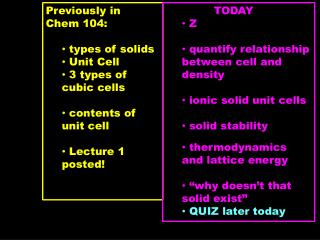# Previously in Chem 104: types of solids Unit Cell 3 types of cubic cells - PowerPoint PPT PresentationDownload PresentationPreviously in Chem 104: types of solids Unit Cell 3 types of cubic cells

Previously in Chem 104: types of solids Unit Cell 3 types of cubic cells
Download Presentation## Previously in Chem 104: types of solids Unit Cell 3 types of cubic cells

- - - - - - - - - - - - - - - - - - - - - - - - - - - E N D - - - - - - - - - - - - - - - - - - - - - - - - - - -
##### Presentation Transcript

1. Previously in • Chem 104: • types of solids • Unit Cell • 3 types of cubic cells • contents of unit cell • Lecture 1 posted! • TODAY • Z • quantify relationship between cell and density • ionic solid unit cells • solid stability • thermodynamics and lattice energy • “why doesn’t that solid exist” • QUIZ later today

2. Three Types of Cubic Unit Cells c b a Body Centered Cubic Face Centered Cubic Simple Cubic

3. What is one result of a metal’s “choice” to adopt a cubic, bcc or fcc lattice? Simple Cubic Body Centered Cubic Face Centered Cubic

4. What is one result of a metal’s “choice” to adopt a cubic, bcc or fcc lattice? Simple Cubic Body Centered Cubic Face Centered Cubic Z = 1 atom/cell Least Dense Z = 2 atom/cell Z = 4 atom/cell Most Dense

5. Simple Cubic Body Centered Cubic Face Centered Cubic Z = 1 Z = 2 Z = 4 Knowing the unit cell structures can be used with other physical data and relationships: Cell volume, V = a3 = l3, l is cell length Cell mass, m = Z x at.wt. A Cell Density = solid density = mass = Z x at.wt. volume A x a3

6. Cell edge, a or cell length, l is related to the atomic radius but depends on which structure: Simple Cubic Body Centered Cubic Face Centered Cubic Z = 1 Z = 2 Z = 4 a = l = 2r

7. Cell edge, a or cell length, l is related to the atomic radius but depends on which structure: Simple Cubic Body Centered Cubic Face Centered Cubic Z = 1 Z = 2 Z = 4 Diagonal 4r = √2a = √2l Solve for edge: 4r /√2 = a = l a = l = 2r a = l = 2√2r

8. Cell edge, a or cell length, l is related to the atomic radius but depends on which structure: Simple Cubic Body Centered Cubic Face Centered Cubic Z = 1 Z = 2 Z = 4 Diagonal 4r a = l = 2r 4r = √3a = √3l a = l = 4r /√3 a = 2.3 r a = l = 2√2r a = 2.8 r

9. Rh metal crystallizes in a cubic lattice where a = 380.34 pm. What is the crystal structure of Rh? Find Z: defines if simple, bcc or fcc Density = Z x at.wt. A x a3 This is a summary of the relationships What do we need? Z What do we have? Nothing here, but can’t we look up Density of Rh metal ? Web Elements: at. weight = 102.91 g/mol Density = 12450 kg m-3 Atomic radius = 173 pm

10. Packing a Square Lattice: Makes a simple cubic cell

11. Can you pack spheres more densely? The Rhomb is the Unit Cell Shape of Hexagonal Lattices

12. Closest Packing: hexagonal layers build up 3D solid

13. Find the triangular gaps in the Pink layer

14. Note how layers “sit” on top of each other: The Cyan layer covers the “up” triangles of the Pink layer The Yellow layer covers the “down” triangles of the Pink layer

15. This packing sequence is ABCA BC, Where B and C cover different “holes” in A A B C A B C

16. Packing direction A B C A B C A C B A C B A ccp Cubic Closest Packing: A B C A B C … Packing direction

17. Packing direction A C B A C B A ccp Cubic Closest Packing: A B C A B C … Packing direction

18. CCP viewed as packing layers CCP viewed unit cell; LOOK! It’s face centered cubic!!! CCP = FCC!! ….mmmMMM C B A C B A

19. Packing direction ABABA . . . . Packed towards you

20. Packing direction A B A B A B A hcp Hexagonal Closest Packing: A B A B … ….mmmMMM Packing direction

21. From Metals to Ionic Solids Will ionic solids pack exactly like metallic solids? Na bcc unit cell as metal NaCl unit cell?

22. From Metals to Ionic Solids • Build up Ionic Solids conceptually like this: • assume Anions are larger than Cations, r- > r+ • pack the Anions into a cubic lattice: ccp, simple or bcc • add Cations to the interstitial spaces (“Mind the gap!”) r- + r+ 2 x r- 2 x r-

23. The Simplest Ionic Solid is CsCl, simple cubic Start with simple cubic Unit cell of Cl- ions Then add one Cs+ in center Z = C. N. (Cs) =

24. How to make NaCl: start with fcc unit cell of Cl- ions